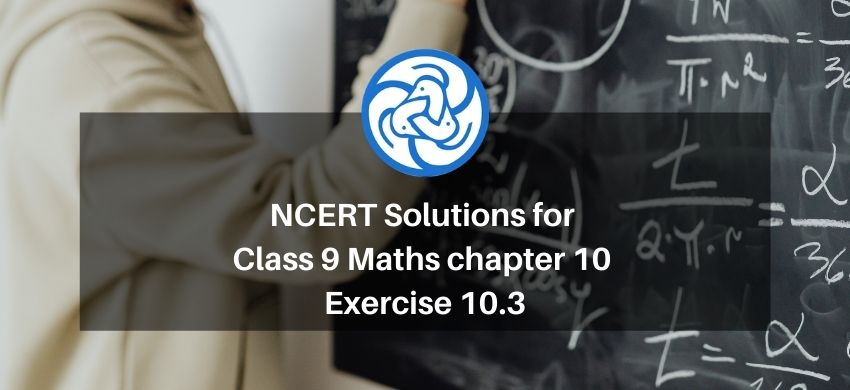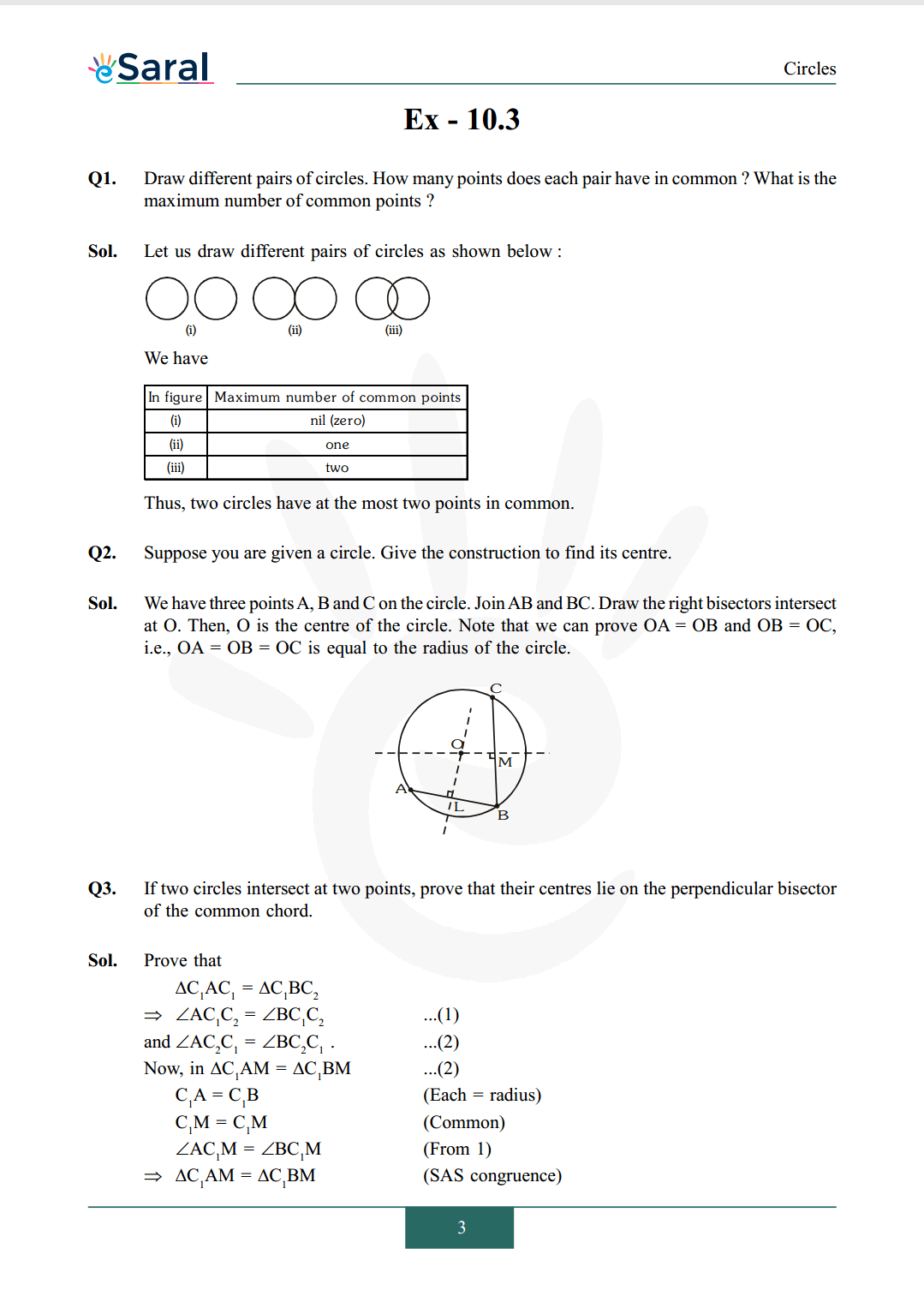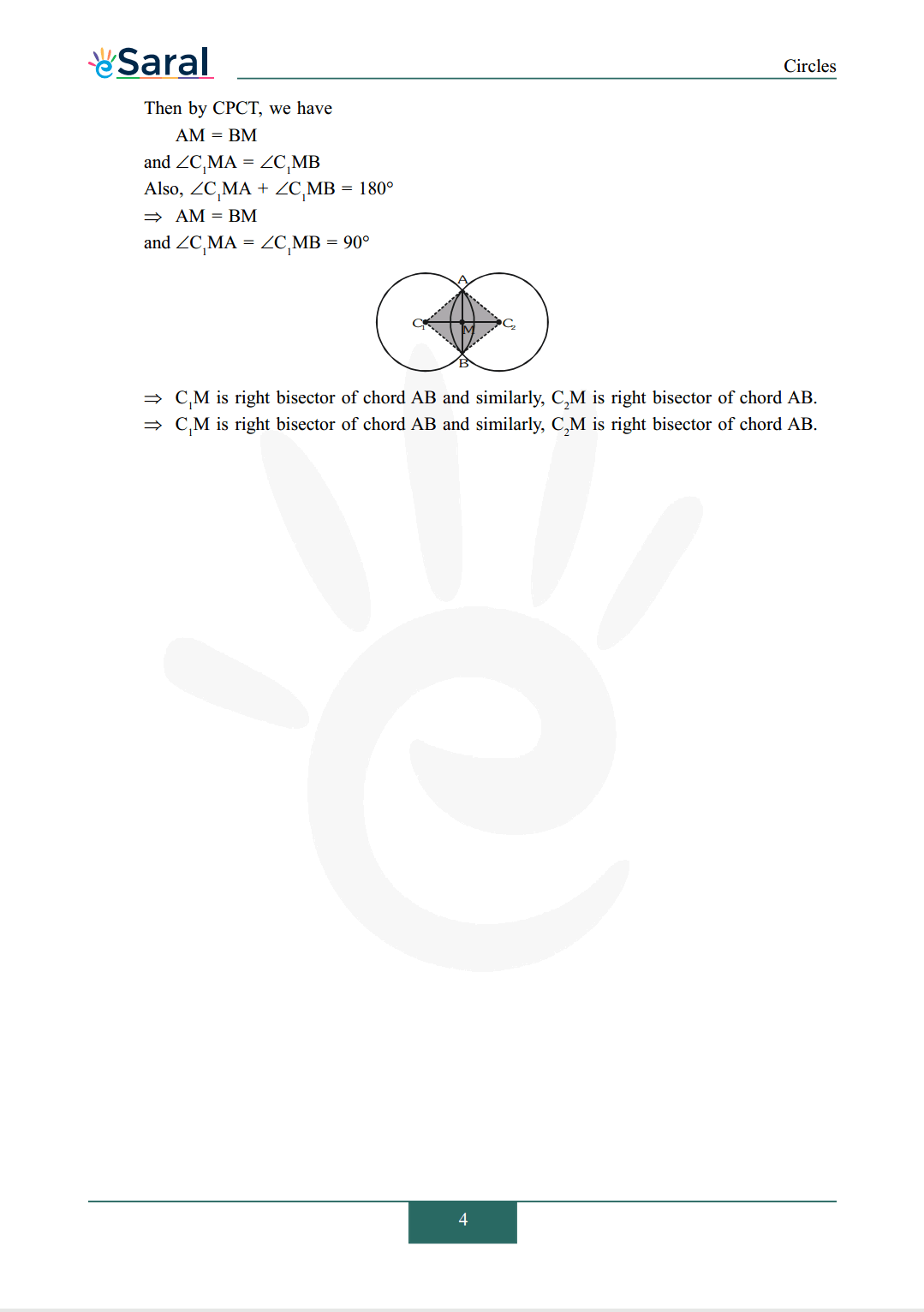Most Affordable JEE | NEET | 8,9,10 Preparation by Kota's Top IITian Doctor Faculties

# NCERT Solutions for Class 9 Maths chapter 10 Exercise 10.3 - Circles - Free PDF Download`
Hey, are you a class 9 Student and Looking for Ways to Download NCERT Solutions for Class 9 Maths chapter 10 Exercise 10.3? If Yes then you are at the right place.

Here we have listed Class 9 maths chapter 10 exercise 10.3 solutions in PDF that is prepared by Kota’s top IITian’s Faculties by keeping Simplicity in mind.

If you want to score high in your class 9 Maths Exam then it is very important for you to have a good knowledge of all the important topics, so to learn and practice those topics you can use eSaral NCERT Solutions.

So, without wasting more time Let’s start.

### Download The PDF of NCERT Solutions for Class 9 Maths chapter 10 Exercise 10.3 "Circles"#### All Questions of Chapter 10 Exercise 10.3

Once you complete the chapter 10 then you can revise Ex. 10.3 by solving following questions

Q1. Draw different pairs of circles. How many points does each pair have in common ? What is the maximum number of common points ?

Q2. Suppose you are given a circle. Give the construction to find its centre.

Q3. If two circles intersect at two points, prove that their centres lie on the perpendicular bisector of the common chord.